Elementary Algebra

# 1.9Properties of Real Numbers

Elementary Algebra1.9 Properties of Real Numbers

### Learning Objectives

By the end of this section, you will be able to:

• Use the commutative and associative properties
• Use the identity and inverse properties of addition and multiplication
• Use the properties of zero
• Simplify expressions using the distributive property

### Be Prepared 1.9

A more thorough introduction to the topics covered in this section can be found in the Prealgebra chapter, The Properties of Real Numbers.

### Use the Commutative and Associative Properties

Think about adding two numbers, say 5 and 3. The order we add them doesn’t affect the result, does it?

$5+33+5885+33+588$
$5+3=3+55+3=3+5$

The results are the same.

As we can see, the order in which we add does not matter!

What about multiplying $5and3?5and3?$

$5·33·515155·33·51515$
$5·3=3·55·3=3·5$

Again, the results are the same!

The order in which we multiply does not matter!

These examples illustrate the commutative property. When adding or multiplying, changing the order gives the same result.

### Commutative Property

$of AdditionIfa,bare real numbers, thena+b=b+aof MultiplicationIfa,bare real numbers, thena·b=b·aof AdditionIfa,bare real numbers, thena+b=b+aof MultiplicationIfa,bare real numbers, thena·b=b·a$

When adding or multiplying, changing the order gives the same result.

The commutative property has to do with order. If you change the order of the numbers when adding or multiplying, the result is the same.

What about subtraction? Does order matter when we subtract numbers? Does $7−37−3$ give the same result as $3−7?3−7?$

$7−33−74−44≠−47−3≠3−77−33−74−44≠−47−3≠3−7$

The results are not the same.

Since changing the order of the subtraction did not give the same result, we know that subtraction is not commutative.

Let’s see what happens when we divide two numbers. Is division commutative?

$12÷44÷121244123133≠1312÷4≠4÷1212÷44÷121244123133≠1312÷4≠4÷12$

The results are not the same.

Since changing the order of the division did not give the same result, division is not commutative. The commutative properties only apply to addition and multiplication!

• Addition and multiplication are commutative.
• Subtraction and Division are not commutative.

If you were asked to simplify this expression, how would you do it and what would your answer be?

$7+8+27+8+2$

Some people would think $7+8is157+8is15$ and then $15+2is17.15+2is17.$ Others might start with $8+2makes108+2makes10$ and then $7+10makes17.7+10makes17.$

Either way gives the same result. Remember, we use parentheses as grouping symbols to indicate which operation should be done first.

 Add $7+87+8$. Add. $(7+8)+2 15+2 17 (7+8)+2 15+2 17$ Add $8+28+2$. Add. $7+(8+2) 7+10 17 7+(8+2) 7+10 17$ $(7+8)+2=7+(8+2)(7+8)+2=7+(8+2)$
Table 1.40

When adding three numbers, changing the grouping of the numbers gives the same result.

This is true for multiplication, too.

 Multiply. $5·135·13$ Multiply. $(5·13)·3 53·3 5 (5·13)·3 53·3 5$ Multiply. $13·313·3$. Multiply. $5·(13·3) 5·1 5 5·(13·3) 5·1 5$ $(5·13)·3=5·(13·3)(5·13)·3=5·(13·3)$
Table 1.41

When multiplying three numbers, changing the grouping of the numbers gives the same result.

You probably know this, but the terminology may be new to you. These examples illustrate the associative property.

### Associative Property

$of AdditionIfa,b,care real numbers, then(a+b)+c=a+(b+c)of MultiplicationIfa,b,care real numbers, then(a·b)·c=a·(b·c)of AdditionIfa,b,care real numbers, then(a+b)+c=a+(b+c)of MultiplicationIfa,b,care real numbers, then(a·b)·c=a·(b·c)$

When adding or multiplying, changing the grouping gives the same result.

Let’s think again about multiplying $5·13·3.5·13·3.$ We got the same result both ways, but which way was easier? Multiplying $1313$ and $33$ first, as shown above on the right side, eliminates the fraction in the first step. Using the associative property can make the math easier!

The associative property has to do with grouping. If we change how the numbers are grouped, the result will be the same. Notice it is the same three numbers in the same order—the only difference is the grouping.

We saw that subtraction and division were not commutative. They are not associative either.

When simplifying an expression, it is always a good idea to plan what the steps will be. In order to combine like terms in the next example, we will use the commutative property of addition to write the like terms together.

### Example 1.122

Simplify: $18p+6q+15p+5q.18p+6q+15p+5q.$

### Try It 1.243

Simplify: $23r+14s+9r+15s.23r+14s+9r+15s.$

### Try It 1.244

Simplify: $37m+21n+4m−15n.37m+21n+4m−15n.$

When we have to simplify algebraic expressions, we can often make the work easier by applying the commutative or associative property first, instead of automatically following the order of operations. When adding or subtracting fractions, combine those with a common denominator first.

### Example 1.123

Simplify: $(513+34)+14.(513+34)+14.$

### Try It 1.245

Simplify: $(715+58)+38.(715+58)+38.$

### Try It 1.246

Simplify: $(29+712)+512.(29+712)+512.$

### Example 1.124

Use the associative property to simplify $6(3x).6(3x).$

### Try It 1.247

Use the associative property to simplify 8(4x).

### Try It 1.248

Use the associative property to simplify $−9(7y).−9(7y).$

### Use the Identity and Inverse Properties of Addition and Multiplication

What happens when we add 0 to any number? Adding 0 doesn’t change the value. For this reason, we call 0 the additive identity.

For example,

$13+0−14+00+(−8)13−14−813+0−14+00+(−8)13−14−8$

These examples illustrate the Identity Property of Addition that states that for any real number $a,a,$ $a+0=aa+0=a$ and $0+a=a.0+a=a.$

What happens when we multiply any number by one? Multiplying by 1 doesn’t change the value. So we call 1 the multiplicative identity.

For example,

$43·1−27·11·3543−273543·1−27·11·3543−2735$

These examples illustrate the Identity Property of Multiplication that states that for any real number $a,a,$ $a·1=aa·1=a$ and $1·a=a.1·a=a.$

We summarize the Identity Properties below.

### Identity Property

$of additionFor any real numbera:a+0=a0+a=a0is theadditive identityof multiplicationFor any real numbera:a·1=a1·a=a1is themultiplicative identityof additionFor any real numbera:a+0=a0+a=a0is theadditive identityof multiplicationFor any real numbera:a·1=a1·a=a1is themultiplicative identity$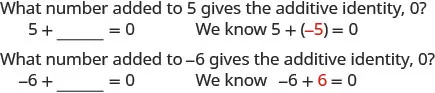Notice that in each case, the missing number was the opposite of the number!

We call $−a.−a.$ the additive inverse of a. The opposite of a number is its additive inverse. A number and its opposite add to zero, which is the additive identity. This leads to the Inverse Property of Addition that states for any real number $a,a+(−a)=0.a,a+(−a)=0.$ Remember, a number and its opposite add to zero.

What number multiplied by $2323$ gives the multiplicative identity, 1? In other words, $2323$ times what results in 1?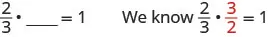What number multiplied by 2 gives the multiplicative identity, 1? In other words 2 times what results in 1?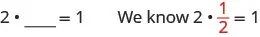Notice that in each case, the missing number was the reciprocal of the number!

We call $1a1a$ the multiplicative inverse of a. The reciprocal of $aa$ number is its multiplicative inverse. A number and its reciprocal multiply to one, which is the multiplicative identity. This leads to the Inverse Property of Multiplication that states that for any real number $a,a≠0,a·1a=1.a,a≠0,a·1a=1.$

We’ll formally state the inverse properties here:

### Inverse Property

 of addition For any real number $aa$,$−a−a$ is the additive inverse of $aa$. A number and its opposite add to zero. $a+(−a)=0a+(−a)=0$ of multiplication For any real number $aa$, $1a1a$ is the multiplicative inverse of $aa$. A number and its reciprocal multiply to one. $a·1a=1a·1a=1$
Table 1.45

### Example 1.125

Find the additive inverse of $5858$ $0.60.6$ $−8−8$ $−43.−43.$

### Try It 1.249

Find the additive inverse of: $7979$ $1.21.2$ $−14−14$ $−94.−94.$

### Try It 1.250

Find the additive inverse of: $713713$ $8.48.4$ $−46−46$ $−52.−52.$

### Example 1.126

Find the multiplicative inverse of $99$ $−19−19$ $0.9.0.9.$

### Try It 1.251

Find the multiplicative inverse of $44$ $−17−17$ $0.30.3$

### Try It 1.252

Find the multiplicative inverse of $1818$ $−45−45$ $0.6.0.6.$

### Use the Properties of Zero

The identity property of addition says that when we add 0 to any number, the result is that same number. What happens when we multiply a number by 0? Multiplying by 0 makes the product equal zero.

### Multiplication by Zero

For any real number a.

$a·0=00·a=0a·0=00·a=0$

The product of any real number and 0 is 0.

What about division involving zero? What is $0÷3?0÷3?$ Think about a real example: If there are no cookies in the cookie jar and 3 people are to share them, how many cookies does each person get? There are no cookies to share, so each person gets 0 cookies. So,

$0÷3=00÷3=0$

We can check division with the related multiplication fact.

$12÷6=2because2·6=12.12÷6=2because2·6=12.$

So we know $0÷3=00÷3=0$ because $0·3=0.0·3=0.$

### Division of Zero

For any real number a, except $0,0,$ $0a=00a=0$ and $0÷a=0.0÷a=0.$

Zero divided by any real number except zero is zero.

Now think about dividing by zero. What is the result of dividing 4 by 0? Think about the related multiplication fact: $4÷0=?4÷0=?$ means $?·0=4.?·0=4.$ Is there a number that multiplied by 0 gives 4? Since any real number multiplied by 0 gives 0, there is no real number that can be multiplied by 0 to obtain 4.

We conclude that there is no answer to $4÷04÷0$ and so we say that division by 0 is undefined.

### Division by Zero

For any real number a, except 0, $a0a0$ and $a÷0a÷0$ are undefined.

Division by zero is undefined.

We summarize the properties of zero below.

### Properties of Zero

Multiplication by Zero: For any real number a,

 $a·0=00·a=0a·0=00·a=0$ The product of any number and 0 is 0.
Table 1.46

Division of Zero, Division by Zero: For any real number $a,a≠0a,a≠0$

 $0a=00a=0$ Zero divided by any real number except itself is zero. $a0is undefineda0is undefined$ Division by zero is undefined.
Table 1.47

### Example 1.127

Simplify: $−8·0−8·0$ $0−20−2$ $−320.−320.$

### Try It 1.253

Simplify: $−14·0−14·0$ $0−60−6$ $−20.−20.$

### Try It 1.254

Simplify: $0(−17)0(−17)$ $0−100−10$ $−50.−50.$

We will now practice using the properties of identities, inverses, and zero to simplify expressions.

### Example 1.128

Simplify: $0n+5,0n+5,$ where $n≠−5n≠−5$ $10−3p0,10−3p0,$ where $10−3p≠0.10−3p≠0.$

### Example 1.129

Simplify: $−84n+(−73n)+84n.−84n+(−73n)+84n.$

### Try It 1.255

Simplify: $−27a+(−48a)+27a.−27a+(−48a)+27a.$

### Try It 1.256

Simplify: $39x+(−92x)+(−39x).39x+(−92x)+(−39x).$

Now we will see how recognizing reciprocals is helpful. Before multiplying left to right, look for reciprocals—their product is 1.

### Example 1.130

Simplify: $715·823·157.715·823·157.$

### Try It 1.257

Simplify: $916·549·169.916·549·169.$

### Try It 1.258

Simplify: $617·1125·176.617·1125·176.$

### Try It 1.259

Simplify: $0m+7,0m+7,$ where $m≠−7m≠−7$ $18−6c0,18−6c0,$ where $18−6c≠0.18−6c≠0.$

### Try It 1.260

Simplify: $0d−4,whered≠40d−4,whered≠4$ $15−4q0,where15−4q≠0.15−4q0,where15−4q≠0.$

### Example 1.131

Simplify: $34·43(6x+12).34·43(6x+12).$

### Try It 1.261

Simplify: $25·52(20y+50).25·52(20y+50).$

### Try It 1.262

Simplify: $38·83(12z+16).38·83(12z+16).$

### Simplify Expressions Using the Distributive Property

Suppose that three friends are going to the movies. They each need $9.25—that’s 9 dollars and 1 quarter—to pay for their tickets. How much money do they need all together? You can think about the dollars separately from the quarters. They need 3 times$9 so $27, and 3 times 1 quarter, so 75 cents. In total, they need$27.75. If you think about doing the math in this way, you are using the distributive property.

### Distributive Property

$Ifa,b,care real numbers, thena(b+c)=ab+acAlso,(b+c)a=ba+caa(b−c)=ab−ac(b−c)a=ba−caIfa,b,care real numbers, thena(b+c)=ab+acAlso,(b+c)a=ba+caa(b−c)=ab−ac(b−c)a=ba−ca$

Back to our friends at the movies, we could find the total amount of money they need like this:

$3(9.25)3(9+0.25)3(9)+3(0.25)27+0.7527.753(9.25)3(9+0.25)3(9)+3(0.25)27+0.7527.75$

In algebra, we use the distributive property to remove parentheses as we simplify expressions.

For example, if we are asked to simplify the expression $3(x+4),3(x+4),$ the order of operations says to work in the parentheses first. But we cannot add x and 4, since they are not like terms. So we use the distributive property, as shown in Example 1.132.

### Example 1.132

Simplify: $3(x+4).3(x+4).$

### Try It 1.263

Simplify: $4(x+2).4(x+2).$

### Try It 1.264

Simplify: $6(x+7).6(x+7).$

Some students find it helpful to draw in arrows to remind them how to use the distributive property. Then the first step in Example 1.132 would look like this: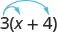### Example 1.133

Simplify: $8(38x+14).8(38x+14).$

### Try It 1.265

Simplify: $6(56y+12).6(56y+12).$

### Try It 1.266

Simplify: $12(13n+34).12(13n+34).$

Using the distributive property as shown in Example 1.134 will be very useful when we solve money applications in later chapters.

### Example 1.134

Simplify: $100(0.3+0.25q).100(0.3+0.25q).$

### Try It 1.267

Simplify: $100(0.7+0.15p).100(0.7+0.15p).$

### Try It 1.268

Simplify: $100(0.04+0.35d).100(0.04+0.35d).$

When we distribute a negative number, we need to be extra careful to get the signs correct!

### Example 1.135

Simplify: $−2(4y+1).−2(4y+1).$

### Try It 1.269

Simplify: $−3(6m+5).−3(6m+5).$

### Try It 1.270

Simplify: $−6(8n+11).−6(8n+11).$

### Example 1.136

Simplify: $−11(4−3a).−11(4−3a).$

### Try It 1.271

Simplify: $−5(2−3a).−5(2−3a).$

### Try It 1.272

Simplify: $−7(8−15y).−7(8−15y).$

Example 1.137 will show how to use the distributive property to find the opposite of an expression.

### Example 1.137

Simplify: $−(y+5).−(y+5).$

### Try It 1.273

Simplify: $−(z−11).−(z−11).$

### Try It 1.274

Simplify: $−(x−4).−(x−4).$

There will be times when we’ll need to use the distributive property as part of the order of operations. Start by looking at the parentheses. If the expression inside the parentheses cannot be simplified, the next step would be multiply using the distributive property, which removes the parentheses. The next two examples will illustrate this.

### Example 1.138

Simplify: $8−2(x+3).8−2(x+3).$

Be sure to follow the order of operations. Multiplication comes before subtraction, so we will distribute the 2 first and then subtract.

### Try It 1.275

Simplify: $9−3(x+2).9−3(x+2).$

### Try It 1.276

Simplify: $7x−5(x+4).7x−5(x+4).$

### Example 1.139

Simplify: $4(x−8)−(x+3).4(x−8)−(x+3).$

### Try It 1.277

Simplify: $6(x−9)−(x+12).6(x−9)−(x+12).$

### Try It 1.278

Simplify: $8(x−1)−(x+5).8(x−1)−(x+5).$

All the properties of real numbers we have used in this chapter are summarized in Table 1.57.

 Commutative Property of addition If $a,ba,b$ are real numbers, then  of multiplication If $a,ba,b$ are real numbers, then $a+b=b+aa+b=b+a$$a·b=b·aa·b=b·a$ Associative Property of addition If $a,b,ca,b,c$ are real numbers, then  of multiplication If $a,b,ca,b,c$ are real numbers, then $(a+b)+c=a+(b+c)(a+b)+c=a+(b+c)$$(a·b)·c=a·(b·c)(a·b)·c=a·(b·c)$ Distributive Property If $a,b,ca,b,c$ are real numbers, then $a(b+c)=ab+aca(b+c)=ab+ac$ Identity Property of addition For any real number $a:a:$    0 is the additive identity  of multiplication For any real number $a:a:$    $11$ is the multiplicative identity $a+0=a0+a=aa+0=a0+a=a$$a·1=a1·a=aa·1=a1·a=a$ Inverse Property of addition For any real number $a,a,$    $−a−a$ is the additive inverse of $aa$  of multiplication For any real number $a,a≠0a,a≠0$    $1a1a$ is the multiplicative inverse of $a.a.$ $a+(−a)=0a+(−a)=0$$a·1a=1a·1a=1$ Properties of Zero For any real number a,  For any real number $a,a≠0a,a≠0$  For any real number $a,a≠0a,a≠0$ $a·0=00·a=0a·0=00·a=0$$0a=00a=0$$a0a0$ is undefined
Table 1.57

### Section 1.9 Exercises

#### Practice Makes Perfect

Use the Commutative and Associative Properties

In the following exercises, use the associative property to simplify.

723.

3(4x)

724.

4(7m)

725.

$( y + 12 ) + 28 ( y + 12 ) + 28$

726.

$( n + 17 ) + 33 ( n + 17 ) + 33$

In the following exercises, simplify.

727.

$1 2 + 7 8 + ( − 1 2 ) 1 2 + 7 8 + ( − 1 2 )$

728.

$2 5 + 5 12 + ( − 2 5 ) 2 5 + 5 12 + ( − 2 5 )$

729.

$3 20 · 49 11 · 20 3 3 20 · 49 11 · 20 3$

730.

$13 18 · 25 7 · 18 13 13 18 · 25 7 · 18 13$

731.

$−24.7 · 3 8 −24.7 · 3 8$

732.

$−36 · 11 · 4 9 −36 · 11 · 4 9$

733.

$( 5 6 + 8 15 ) + 7 15 ( 5 6 + 8 15 ) + 7 15$

734.

$( 11 12 + 4 9 ) + 5 9 ( 11 12 + 4 9 ) + 5 9$

735.

17(0.25)(4)

736.

36(0.2)(5)

737.

[2.48(12)](0.5)

738.

[9.731(4)](0.75)

739.

7(4a)

740.

9(8w)

741.

$−15 ( 5 m ) −15 ( 5 m )$

742.

$−23 ( 2 n ) −23 ( 2 n )$

743.

$12 ( 5 6 p ) 12 ( 5 6 p )$

744.

$20 ( 3 5 q ) 20 ( 3 5 q )$

745.

$43 m + ( −12 n ) + ( −16 m ) + ( −9 n ) 43 m + ( −12 n ) + ( −16 m ) + ( −9 n )$

746.

$−22 p + 17 q + ( −35 p ) + ( −27 q ) −22 p + 17 q + ( −35 p ) + ( −27 q )$

747.

$3 8 g + 1 12 h + 7 8 g + 5 12 h 3 8 g + 1 12 h + 7 8 g + 5 12 h$

748.

$5 6 a + 3 10 b + 1 6 a + 9 10 b 5 6 a + 3 10 b + 1 6 a + 9 10 b$

749.

$6.8 p + 9.14 q + ( −4.37 p ) + ( −0.88 q ) 6.8 p + 9.14 q + ( −4.37 p ) + ( −0.88 q )$

750.

$9.6 m + 7.22 n + ( −2.19 m ) + ( −0.65 n ) 9.6 m + 7.22 n + ( −2.19 m ) + ( −0.65 n )$

Use the Identity and Inverse Properties of Addition and Multiplication

In the following exercises, find the additive inverse of each number.

751.

$2525$
4.3
$−8−8$
$−103−103$

752.

$5959$
2.1
$−3−3$
$−95−95$

753.

$−76−76$
$−0.075−0.075$
23
$1414$

754.

$−83−83$
$−0.019−0.019$
52
$5656$

In the following exercises, find the multiplicative inverse of each number.

755.

6 $−34−34$ 0.7

756.

12 $−92−92$ 0.13

757.

$11121112$ $−1.1−1.1$ $−4−4$

758.

$17201720$ $−1.5−1.5$ $−3−3$

Use the Properties of Zero

In the following exercises, simplify.

759.

$0 6 0 6$

760.

$3 0 3 0$

761.

$0 ÷ 11 12 0 ÷ 11 12$

762.

$6 0 6 0$

763.

$0 3 0 3$

764.

$0 · 8 15 0 · 8 15$

765.

$( −3.14 ) ( 0 ) ( −3.14 ) ( 0 )$

766.

$1 10 0 1 10 0$

Mixed Practice

In the following exercises, simplify.

767.

$19 a + 44 − 19 a 19 a + 44 − 19 a$

768.

$27 c + 16 − 27 c 27 c + 16 − 27 c$

769.

$10 ( 0.1 d ) 10 ( 0.1 d )$

770.

$100 ( 0.01 p ) 100 ( 0.01 p )$

771.

$0u−4.99,0u−4.99,$ where $u≠4.99u≠4.99$

772.

$0v−65.1,0v−65.1,$ where $v≠65.1v≠65.1$

773.

$0÷(x−12),0÷(x−12),$ where $x≠12x≠12$

774.

$0÷(y−16),0÷(y−16),$ where $x≠16x≠16$

775.

$32−5a0,32−5a0,$ where
$32−5a≠032−5a≠0$

776.

$28−9b0,28−9b0,$ where
$28−9b≠028−9b≠0$

777.

$(34+910m)÷0(34+910m)÷0$ where
$34+910m≠034+910m≠0$

778.

$(516n−37)÷0(516n−37)÷0$ where
$516n−37≠0516n−37≠0$

779.

$15 · 3 5 ( 4 d + 10 ) 15 · 3 5 ( 4 d + 10 )$

780.

$18 · 5 6 ( 15 h + 24 ) 18 · 5 6 ( 15 h + 24 )$

Simplify Expressions Using the Distributive Property

In the following exercises, simplify using the distributive property.

781.

$8 ( 4 y + 9 ) 8 ( 4 y + 9 )$

782.

$9 ( 3 w + 7 ) 9 ( 3 w + 7 )$

783.

$6 ( c − 13 ) 6 ( c − 13 )$

784.

$7 ( y − 13 ) 7 ( y − 13 )$

785.

$1 4 ( 3 q + 12 ) 1 4 ( 3 q + 12 )$

786.

$1 5 ( 4 m + 20 ) 1 5 ( 4 m + 20 )$

787.

$9 ( 5 9 y − 1 3 ) 9 ( 5 9 y − 1 3 )$

788.

$10 ( 3 10 x − 2 5 ) 10 ( 3 10 x − 2 5 )$

789.

$12 ( 1 4 + 2 3 r ) 12 ( 1 4 + 2 3 r )$

790.

$12 ( 1 6 + 3 4 s ) 12 ( 1 6 + 3 4 s )$

791.

$r ( s − 18 ) r ( s − 18 )$

792.

$u ( v − 10 ) u ( v − 10 )$

793.

$( y + 4 ) p ( y + 4 ) p$

794.

$( a + 7 ) x ( a + 7 ) x$

795.

$−7 ( 4 p + 1 ) −7 ( 4 p + 1 )$

796.

$−9 ( 9 a + 4 ) −9 ( 9 a + 4 )$

797.

$−3 ( x − 6 ) −3 ( x − 6 )$

798.

$−4 ( q − 7 ) −4 ( q − 7 )$

799.

$− ( 3 x − 7 ) − ( 3 x − 7 )$

800.

$− ( 5 p − 4 ) − ( 5 p − 4 )$

801.

$16 − 3 ( y + 8 ) 16 − 3 ( y + 8 )$

802.

$18 − 4 ( x + 2 ) 18 − 4 ( x + 2 )$

803.

$4 − 11 ( 3 c − 2 ) 4 − 11 ( 3 c − 2 )$

804.

$9 − 6 ( 7 n − 5 ) 9 − 6 ( 7 n − 5 )$

805.

$22 − ( a + 3 ) 22 − ( a + 3 )$

806.

$8 − ( r − 7 ) 8 − ( r − 7 )$

807.

$( 5 m − 3 ) − ( m + 7 ) ( 5 m − 3 ) − ( m + 7 )$

808.

$( 4 y − 1 ) − ( y − 2 ) ( 4 y − 1 ) − ( y − 2 )$

809.

$5 ( 2 n + 9 ) + 12 ( n − 3 ) 5 ( 2 n + 9 ) + 12 ( n − 3 )$

810.

$9 ( 5 u + 8 ) + 2 ( u − 6 ) 9 ( 5 u + 8 ) + 2 ( u − 6 )$

811.

$9 ( 8 x − 3 ) − ( −2 ) 9 ( 8 x − 3 ) − ( −2 )$

812.

$4 ( 6 x − 1 ) − ( −8 ) 4 ( 6 x − 1 ) − ( −8 )$

813.

$14 ( c − 1 ) − 8 ( c − 6 ) 14 ( c − 1 ) − 8 ( c − 6 )$

814.

$11 ( n − 7 ) − 5 ( n − 1 ) 11 ( n − 7 ) − 5 ( n − 1 )$

815.

$6 ( 7 y + 8 ) − ( 30 y − 15 ) 6 ( 7 y + 8 ) − ( 30 y − 15 )$

816.

$7 ( 3 n + 9 ) − ( 4 n − 13 ) 7 ( 3 n + 9 ) − ( 4 n − 13 )$

#### Everyday Math

817.

Insurance copayment Carrie had to have 5 fillings done. Each filling cost $80. Her dental insurance required her to pay 20% of the cost as a copay. Calculate Carrie’s copay: 1. First, by multiplying 0.20 by 80 to find her copay for each filling and then multiplying your answer by 5 to find her total copay for 5 fillings. 2. Next, by multiplying [5(0.20)](80) 3. Which of the properties of real numbers says that your answers to parts (a), where you multiplied 5[(0.20)(80)] and (b), where you multiplied [5(0.20)](80), should be equal? 818. Cooking time Helen bought a 24-pound turkey for her family’s Thanksgiving dinner and wants to know what time to put the turkey in to the oven. She wants to allow 20 minutes per pound cooking time. Calculate the length of time needed to roast the turkey: 1. First, by multiplying $24·2024·20$ to find the total number of minutes and then multiplying the answer by $160160$ to convert minutes into hours. 2. Next, by multiplying $24(20·160).24(20·160).$ 3. Which of the properties of real numbers says that your answers to parts (a), where you multiplied $(24·20)160,(24·20)160,$ and (b), where you multiplied $24(20·160),24(20·160),$ should be equal? 819. Buying by the case Trader Joe’s grocery stores sold a bottle of wine they called “Two Buck Chuck” for$1.99. They sold a case of 12 bottles for $23.88. To find the cost of 12 bottles at$1.99, notice that 1.99 is $2−0.01.2−0.01.$

1. Multiply 12(1.99) by using the distributive property to multiply $12(2−0.01).12(2−0.01).$
2. Was it a bargain to buy “Two Buck Chuck” by the case?
820.

#### Self Check

After completing the exercises, use this checklist to evaluate your mastery of the objectives of this section.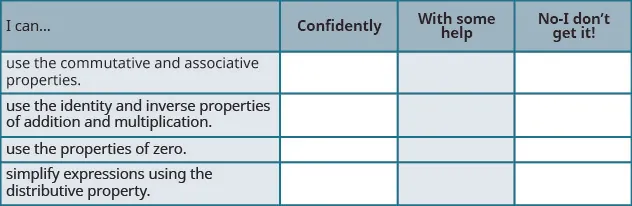After reviewing this checklist, what will you do to become confident for all objectives?

Order a print copy

As an Amazon Associate we earn from qualifying purchases.